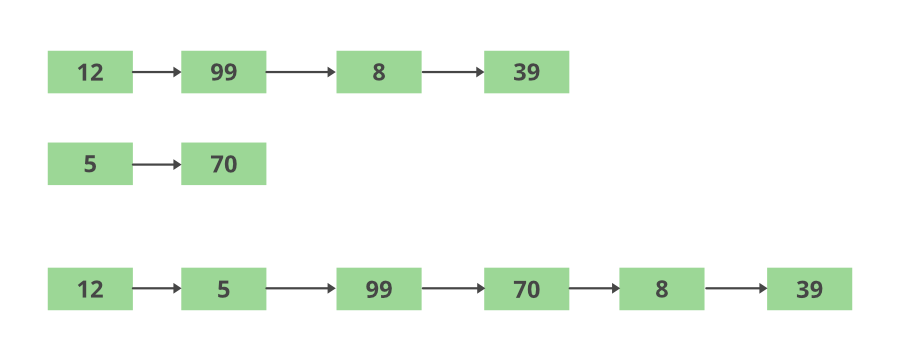Open in App
Not now

# Java Program to Combine Two List by Alternatively Taking Elements

• Last Updated : 08 Aug, 2022

A list is an ordered sequence of elements stored together to form a collection. A list can contain duplicate as well as null entries. A list allows us to perform index-based operations, that is additions, deletions, manipulations, and positional access. Java provides an in-built interface <<java.util>> to perform list as well as other class-based functions.

Cases: There can occur two different scenarios while doing so as per the length of lists

1. If list 2 gets exhausted while adding elements alternatively then the remaining elements of list 1 are the second list is remaining elements of list 1 to be added in the same sequence of occurrence.
2. If list 1 gets exhausted and so on as discussed in the above case vice-versa

So, the Aim is to completely remove the elements from second one and add to first one list and whatever is left will be the second list.Approach: The following approach is adopted to store elements alternatively in a merged list.

• Two lists are declared and initialized with a set of elements.
• Two counters, i and j are maintained to iterate over the length of the lists. The loop runs until the shorter length of both the lists.
• An empty list is maintained to store the merged contents of both the lists, in order of list1 followed by list2.
• At the end of the loop, one of the list, that is a shorter one is exhausted. A loop is then used to iterate over the remaining elements of the longer list and store them at the end one by one.
• The data type of the merged list should be similar to the individual lists.

Implementation: Two examples are discussed below considering both integer list and string lists

Example 1: String Lists

## Java

 `// Java Program to Combine Two List``// by Alternatingly Taking Elements` `// importing required packages``import` `java.io.*;``import` `java.util.*;``import` `java.util.Iterator;` `// Class to access alternate elements``class` `GFG {` `    ``// Main driver method``    ``public` `static` `void` `main(String[] args)``    ``{` `        ``// Creating(declaring) list1``        ``List list1 = ``new` `ArrayList();``        ``// Adding elements to list1``        ``// Custom inputs``        ``list1.add(``"Geeks"``);``        ``list1.add(``"Geeks"``);``        ``list1.add(``"portal"``);` `        ``// Creating(declaring) list2``        ``List list2 = ``new` `ArrayList();` `        ``// Adding elements to list2``        ``// Custom inputs``        ``list2.add(``"for"``);``        ``list2.add(``"is CSE"``);``        ``list2.add(``"portal"``);` `        ``// Display message``        ``System.out.print(``"List1 contents: "``);` `        ``// Iterating over List1``        ``Iterator iterator = list1.iterator();` `        ``// Condition check using hasNext() which holds true``        ``// till there is single element remaining in the``        ``// List``        ``while` `(iterator.hasNext()) {` `            ``// Printing elements of List 1``            ``System.out.print(iterator.next() + ``" "``);``        ``}` `        ``// Next Line``        ``System.out.println();` `        ``// Display message``        ``System.out.print(``"List2 contents: "``);` `        ``// Iterating over List 2``        ``iterator = list2.iterator();` `        ``// Condition check using hasNext() which holds true``        ``// till there is single element remaining in the``        ``// List``        ``while` `(iterator.hasNext()) {` `            ``// Printing elements of List 2``            ``System.out.print(iterator.next() + ``" "``);``        ``}` `        ``// Declaring counters``        ``int` `i = ``0``;``        ``int` `j = ``0``;` `        ``// Creating(declaring) merged List``        ``List merged_list = ``new` `ArrayList();` `        ``// Iterating over both the lists until``        ``// the elements of shorter List are exhausted``        ``while` `(i < list1.size() && j < list2.size()) {` `            ``// Step 1: Adding List1 element``            ``merged_list.add(list1.get(i));` `            ``// Step 2: Adding List2 element``            ``merged_list.add(list2.get(j));` `            ``// Incrementing counters``            ``i++;``            ``j++;``        ``}` `        ``// Iterating over the remaining part of List1``        ``while` `(i < list1.size()) {``            ``merged_list.add(list1.get(i));` `            ``// Incrementing List1 counter``            ``i++;``        ``}` `        ``// Iterating over the remaining part of List2``        ``while` `(j < list2.size()) {``            ``merged_list.add(list2.get(j));` `            ``// Incrementing List1 counter``            ``j++;``        ``}` `        ``// Next line``        ``System.out.println();` `        ``// Display message``        ``System.out.print(``"Merged List contents: "``);` `        ``// Iterators``        ``iterator = merged_list.iterator();` `        ``// Iterating over merged List using hasNext() method``        ``// which holds true till there is single element``        ``// remaining``        ``while` `(iterator.hasNext()) {` `            ``// Printing merged list contents``            ``System.out.print(iterator.next() + ``" "``);``        ``}``    ``}``}`

Output

```List1 contents: Geeks Geeks portal
List2 contents: for is CSE portal
Merged List contents: Geeks for Geeks is CSE portal portal ```

Example 2: Integer Lists

## Java

 `// Java Program to Combine Two List``// by Alternatingly Taking Elements` `// importing required packages``import` `java.io.*;``import` `java.util.*;``import` `java.util.Iterator;` `// Class``class` `GFG {` `    ``// Main driver method``    ``public` `static` `void` `main(String[] args)``    ``{``        ``// Creating(declaring) List1``        ``List list1 = ``new` `ArrayList();``        ``// Adding elements to List1``        ``// Custom inputs``        ``list1.add(``2``);``        ``list1.add(``4``);``        ``list1.add(``6``);` `        ``// Creating(declaring) List2``        ``List list2 = ``new` `ArrayList();` `        ``// Adding elements to List2``        ``// Custom inputs``        ``list2.add(``1``);``        ``list2.add(``3``);``        ``list2.add(``5``);``        ``list2.add(``7``);` `        ``// Display message``        ``System.out.print(``"List1 contents: "``);` `        ``// Iterating over List1``        ``Iterator iterator = list1.iterator();` `        ``// ConditionCheck using hasNext() method which hold``        ``// true till single element in remaining List``        ``while` `(iterator.hasNext()) {` `            ``// Printing List1 contents``            ``System.out.print(iterator.next() + ``" "``);``        ``}` `        ``// New line``        ``System.out.println();` `        ``// Display message``        ``System.out.print(``"List2 contents: "``);``        ``iterator = list2.iterator();` `        ``// ConditionCheck using hasNext() method which hold``        ``// true till single element in remaining List``        ``while` `(iterator.hasNext()) {` `            ``// Printing List2 contents``            ``System.out.print(iterator.next() + ``" "``);``        ``}``        ``// Setting counters to zeros``        ``int` `i = ``0``;``        ``int` `j = ``0``;` `        ``// Creating(declaring) merged list``        ``List merged_list``            ``= ``new` `ArrayList();` `        ``// Iterating over both the lists``        ``// until the shorter list``        ``while` `(i < list1.size() && j < list2.size()) {` `            ``// Step 1: Adding List2 element``            ``merged_list.add(list2.get(j));` `            ``// Step 2: Adding List1 element``            ``merged_list.add(list1.get(i));` `            ``// Incrementing counters``            ``i++;``            ``j++;``        ``}` `        ``// Iterating over the remaining part of List1` `        ``// Case 1: Input: ShorterList following BiggerList``        ``while` `(i < list1.size()) {` `            ``// Merge remaining List to List1, and``            ``// making List2 final as NULL List``            ``merged_list.add(list1.get(i));``            ``i++;``        ``}` `        ``// Case 2: Input: BiggerList following ShorterList``        ``while` `(j < list2.size()) {` `            ``// Merge remaining List to List1,an d``            ``// making List2 -> NULL List``            ``merged_list.add(list2.get(j));``            ``j++;``        ``}` `        ``// New line``        ``System.out.println();` `        ``// Display message``        ``System.out.print(``"Merged List contents: "``);` `        ``// Iterating over merged list``        ``iterator = merged_list.iterator();` `        ``// Condition check using hasNext() method which``        ``// holds true till there is single element remaining``        ``// in the List``        ``while` `(iterator.hasNext()) {` `            ``// Printing merged List contents i.e``            ``// FinalList = List1 + List2(Null final List)``            ``System.out.print(iterator.next() + ``" "``);``        ``}``    ``}``}`

Output

```List1 contents: 2 4 6
List2 contents: 1 3 5 7
Merged List contents: 1 2 3 4 5 6 7 ```

My Personal Notes arrow_drop_up